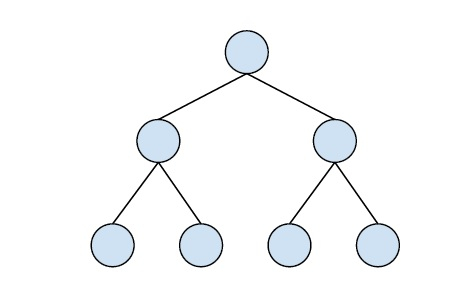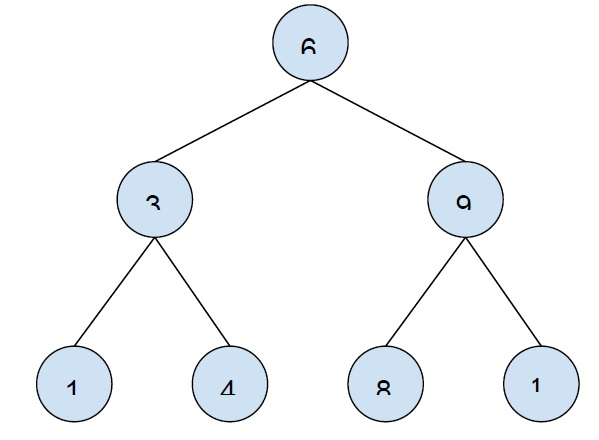# Print all odd nodes of Binary Search Tree in C++

In this problem, we are given a binary search tree and we have to print all the nodes that have odd values.

The binary search tree is a special type of tree that possess the following properties −

• The left subtree always has values smaller than the root node.

• The right subtree always has values larger than the root node.

• Both the left and right subtree should also follow the above two properties.Let’s take an example to understand the problem −Output − 1 3 9

To solve this problem, a simple approach would be traversing the tree. On traversal, we will check the value of each node of the tree. If the node is odd print it otherwise more to the next node of the tree.

The complexity of the program will depend on the number of nodes. Time complexity: O(n).

## Example

The below program shows the implementation of our solution −

Live Demo

#include <bits/stdc++.h>
using namespace std;
struct Node {
int key;
struct Node *left, *right;
};
Node* newNode(int item){
Node* temp = new Node;
temp->key = item;
temp->left = temp->right = NULL;
return temp;
}
Node* insertNode(Node* node, int key){
if (node == NULL)
return newNode(key);
if (key < node->key)
node->left = insertNode(node->left, key);
else
node->right = insertNode(node->right, key);
return node;
}
void printOddNodes(Node* root){
if (root != NULL) {
printOddNodes(root->left);
if (root->key % 2 != 0)
cout<<root->key<<"\t";
printOddNodes(root->right);
}
}
int main(){
Node* root = NULL;
root = insertNode(root, 6);
root = insertNode(root, 3);
root = insertNode(root, 1);
root = insertNode(root, 4);
root = insertNode(root, 9);
root = insertNode(root, 8);
root = insertNode(root, 10);
cout<<"Nodes with odd values are :\n";
printOddNodes(root);
return 0;
}

## Output

Nodes with odd values are −

1 3 9